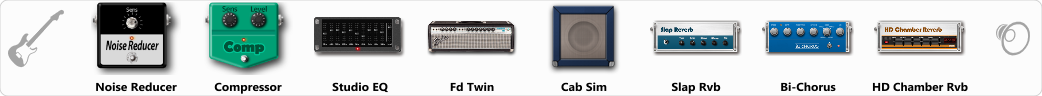# SowndGod

Discussion in 'ToneLib-GFX presets' started by Jean C.Guion, Sep 16, 2019.

1. SowndGod

Preset name: Big choursy studio twin[brite]

monster clean tone

Effects chain:Effect: "Noise Reducer" (Dynamics / Filter), active - "yes"
"Sens" = 28
"Mode" = Soft

Effect: "Compressor" (Dynamics / Filter), active - "yes"
"Sense" = 1
"Level" = 85

Effect: "Studio EQ" (Dynamics / Filter), active - "yes"
"31 Hz" = -15
"62 Hz" = -6
"125 Hz" = 5
"250 Hz" = 6
"500 Hz" = 4
"1 kHz" = 5
"2 kHz" = -3
"4 kHz" = 2
"8 kHz" = -2
"16 kHz" = 5
"above 16 kHz" = 0
"Level (dB)" = 7

Effect: "Fd Twin" (Amp simulators), active - "yes"
"Gain" = 17
"Bass" = 94
"Middle" = 62
"Treble" = 48
"Presence" = 19
"Master" = 55
"Output" = 50
"Level (dB)" = 15

Effect: "Cab Sim" (Cabinets), active - "yes"
"Model" = 4x12" Greenback
"Level (dB)" = -2

Effect: "Slap Rvb" (Reverb), active - "yes"
"Time" = 6.3
"PreDelay" = 0
"LoDamp" = 18
"HiDamp" = 40
"Mix" = 41

Effect: "Bi-Chorus" (Modulation / Sfx), active - "yes"
"Speed A" = 3.2
"Speed B" = 4.0
"Depth" = 43
"Reso" = 0
"Mode" = Parallel
"Mix" = 56

Effect: "HD Chamber Rvb" (Reverb), active - "yes"
"Time" = 8.2
"PreLPF" = 79
"PreDelay" = 61
"HiDamp" = 21
"LoGain" = -2.1
"Mix" = 37

Note: You will need to download and install the ToneLib-GFX software to use the preset.

File size:
707 bytes
Views:
3,198